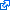# 1 paper accepted to TAC

Our paper on linear-convex optimal steady-state control  has been accepted to IEEE Transactions on Automatic Control!L. S. P. Lawrence, J. W. Simpson-Porco, and E. Mallada, “Linear-Convex Optimal Steady-State Control,” IEEE Transactions on Automatic Control, pp. 5377-5385, 2021.

We consider the problem of designing a feedback controller for a multivariable nonlinear system that regulates an arbitrary subset of the system states and inputs to the solution of a constrained optimization problem, despite parametric modelling uncertainty and time-varying exogenous disturbances; we term this the optimal steady-state (OSS) control problem. We derive necessary and sufficient conditions for the existence of an OSS controller by formulating the OSS control problem as an output regulation problem wherein the regulation error is unmeasurable. We introduce the notion of an optimality model, and show that the existence of an optimality model is sufficient to reduce the OSS control problem to an output regulation problem with measurable error. This yields a design framework for OSS control that unifies and extends many existing designs in the literature. We present a complete and constructive solution of the OSS control problem for the case where the plant is linear time-invariant with structured parametric uncertainty, and disturbances are constant in time. We illustrate these results via an application to optimal frequency control of power networks, and show that our design procedure recovers several frequency controllers from the recent literature.

``````@article{lsm2020tac,
abstract = {We consider the problem of designing a feedback controller for a multivariable nonlinear system that regulates an arbitrary subset of the system states and inputs to the solution of a constrained optimization problem, despite parametric modelling uncertainty and time-varying exogenous disturbances; we term this the optimal steady-state (OSS) control problem. We derive necessary and sufficient conditions for the existence of an OSS controller by formulating the OSS control problem as an output regulation problem wherein the regulation error is unmeasurable. We introduce the notion of an optimality model, and show that the existence of an optimality model is sufficient to reduce the OSS control problem to an output regulation  problem with measurable error. This yields a design framework for OSS control that unifies and extends many existing designs in the literature. We present a complete and constructive solution of the OSS control problem for the case where the plant is linear time-invariant with structured parametric uncertainty, and disturbances are constant in time. We illustrate these results via an application to optimal frequency control of power networks, and show that our design procedure recovers several frequency controllers from the recent literature.},
author = {Lawrence, Liam S. P. and Simpson-Porco, John W. and Mallada, Enrique},
doi = {10.1109/TAC.2020.3044275},
grants = {CAREER-1752362;TRIPODS-1934979;CPS-2136324},
journal = {IEEE Transactions on Automatic Control},
month = {11},
pages = {5377-5385},
record = {early access Dec 2020, accepted Nov 2020, conditionally accepted Aug. 2020, 2nd revision May 2020, revised Sept 2019, submitted Oct. 2018},
title = {Linear-Convex Optimal Steady-State Control},